# Difference between revisions of "Infinite game"

A non-cooperative game, in particular a two-person zero-sum game, with infinite sets of player strategies. Let

\$\$\Gamma = (X_1,X_2,...,X_n,H_1,H_2,...,H_n)\$\$

be an infinite game withparticipants. It was shown by C. Berge  that ifare locally convex compact linear topological Hausdorff spaces, if the pay-off functions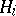are continuous onand are quasi-concave for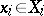,, then the gamehas equilibrium points (solutions). It was also shown  that if the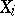are compact Hausdorff spaces and theare continuous on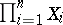,, thenhas equilibrium points in mixed strategies. However, not all infinite games have equilibrium points, even in mixed strategies. For example, for the two-person zero-sum game in which the sets of player strategies are sets of integers, while the pay-off function has the form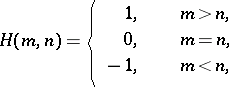no value exists. The best studied classes of infinite games in normal form are infinite two-person zero-sum games and, in particular, games on the unit square (cf. Game on the unit square).

How to Cite This Entry:
Infinite game. Encyclopedia of Mathematics. URL: http://encyclopediaofmath.org/index.php?title=Infinite_game&oldid=28796
This article was adapted from an original article by E.B. Yanovskaya (originator), which appeared in Encyclopedia of Mathematics - ISBN 1402006098. See original article